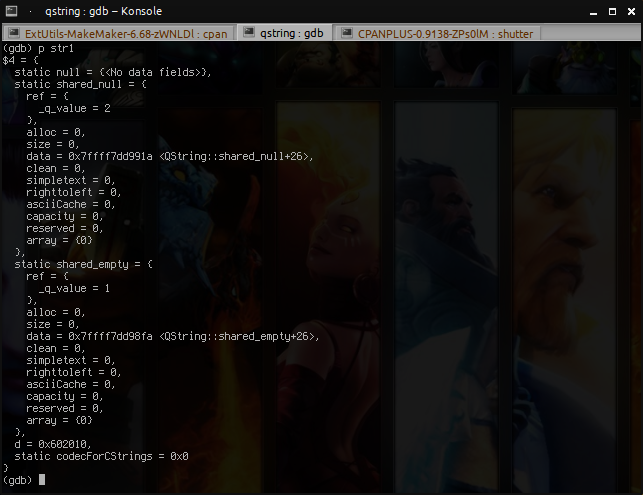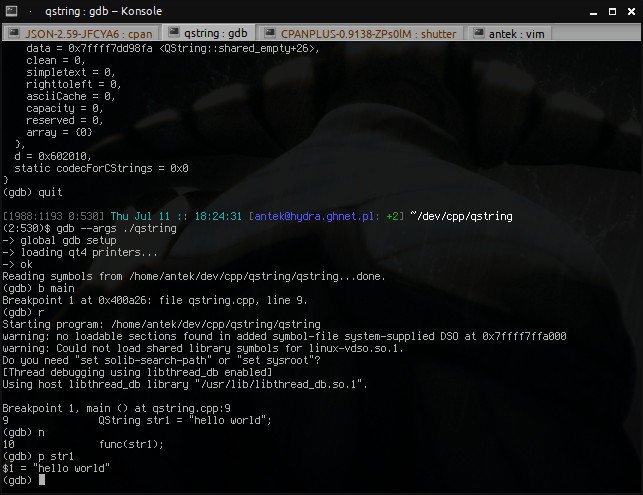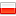### Friendly GDB

Thu, 11 July 2013 :: #gdb :: #linux

The `gdb` debugger is a very old application, used widely in the past, when a computer was not yet a part of every house's inventory. Contrary to what many people say, it's very usable even today, mainly because of its extensibility, which lets the user to adapt it to his/hers specific needs.

It's quite easy to add custom renderers to `gdb`. They are a piece of code that convert data types into human readable form, so it's easy to understand given type by just looking at the data dump.

Here is an example data dump of the `QString` structure (it's a container for strings in the Qt Framework), created by using the `print` command:As you can see, you can't see much ;). Maybe this kind of information is useful for a Qt developer, which is debugging the functionality of `QString` structure, but our case is completely different. The solution for this problem is to tell `gdb` what kind of information we seek and make it display only these fields which match our interest.

The first step is to acquire the Qt renderer pack. You can get it from i.e. KDevelop's repository. It was written by Niko Sams, and it definitely does the job well. We have to use the `qt4.py` file, which contains the code to convert raw structure dump into more eye-friendly notation. As you can also probably see, the same directory in the repository also contains another file named `libstdcxx.py` which does the same thing for classes from `std` library (standard C++ library), like `std::string`, `std::map`, and others. These files can be copied into any directory. For example, here seems to be a good spot for it: `/usr/share/gdb/auto-load/usr/lib`.

Step two is to create the `~/.gdbinit` file. It will be automatically loaded during every invocation of `gdb`. Here's what you can put into it:

``````python
import sys

from qt4 import register_qt4_printers
from libstdcxx import register_libstdcxx_printers
register_qt4_printers(None)
register_libstdcxx_printers(None)
end

set print pretty 1
``````

If you'll get errors after invoking `gdb`, you may want to check if:

1. Your path is correct, contains both `.py` files (`qt4.py` and `libstdcxx.py`),
2. The python interpreter is correctly configured (is it v2.7? or maybe v3.0?),
3. You can also review your security settings related to initialization script path whitelisting ({SafePath}[related link]).

If no errors will occur, the next time you will use `print` command on a supported data type, it will display something that is more friendly than ever before:Much better! Other examples:

## Example 1

Without printers:

``````(gdb) p strings
\$1 = {
_M_t = {
_M_impl = {
<std::allocator<std::_Rb_tree_node<std::pair<QString const, int> > >> = {
<__gnu_cxx::new_allocator<std::_Rb_tree_node<std::pair<QString const, int> > >> = {<No data fields>}, <No data fields>},
members of std::_Rb_tree<QString, std::pair<QString const, int>, std::_Select1st<std::pair<QString const, int> >, std::less<QString>, std::allocator<std::pair<QString const, int> > >::_Rb_tree_impl<std::less<QString>, false>:
_M_key_compare = {
<std::binary_function<QString, QString, bool>> = {<No data fields>}, <No data fields>},
_M_color = std::_S_red,
_M_parent = 0x6040c0,
_M_left = 0x604050,
_M_right = 0x604140
},
_M_node_count = 3
}
}
}
``````

With printers:

``````(gdb) p strings
\$1 = std::map with 3 elements = {
["hello world"] = 1,
["test"] = 2,
["test2"] = 3
}
``````

## Example 2

Without printers:

``````(gdb) p v
\$1 = {
<std::_Vector_base<int, std::allocator<int> >> = {
_M_impl = {
<std::allocator<int>> = {
<__gnu_cxx::new_allocator<int>> = {<No data fields>}, <No data fields>},
members of std::_Vector_base<int, std::allocator<int> >::_Vector_impl:
_M_start = 0x6051c0,
_M_finish = 0x6051cc,
_M_end_of_storage = 0x6051d0
}
}, <No data fields>}
``````

With printers:

``````(gdb) p v
\$1 = std::vector of length 3, capacity 4 = {1, 2, 3}
``````

## Example 3

Without printers:

``````(gdb) p strmap
\$1 = {
{
d = 0x6061f0,
e = 0x6061f0
}
}
``````

With printers:

``````(gdb) p strmap
\$1 = QMap<std::basic_string<char, std::char_traits<char>, std::allocator<char> >, std::basic_string<char, std::char_traits<char>, std::allocator<char> >> = {
["a"] = "b",
["b"] = "c",
["c"] = "d"
}
``````

## Conclusion

Okay, the last part could be better (more condensed), but having the access to the source code you can just fix it for yourself. And still it's better than the un-friendly version!